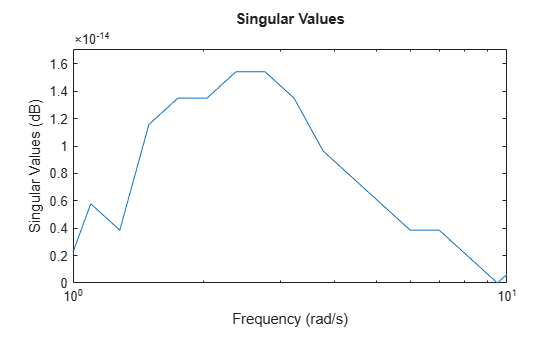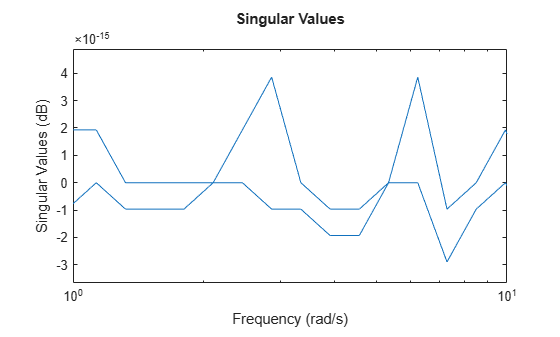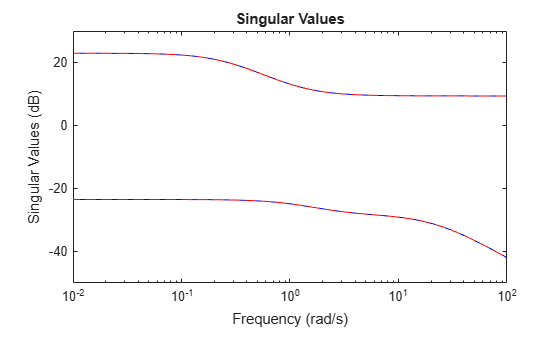# rncf

Right normalized coprime factorization

## Syntax

``fact = rncf(sys)``
``[fact,Mr,Nr] = rncf(sys)``

## Description

example

````fact = rncf(sys)` computes the right normalized coprime factorization of the dynamic system model `sys`. The factorization is given by:$sys={N}_{r}{M}_{r}^{-1},\text{ }{M}_{r}^{*}{M}_{r}+{N}_{r}^{*}{N}_{r}=I.$Here, ${M}_{r}^{*}$ denotes the conjugate of Mr (see `ctranspose`). The returned model `fact` is a minimal state-space realization of the stable system [Mr;Nr]. This factorization is used in other normalized coprime factor computations such as model reduction (`ncfmr`) and controller synthesis (`ncfsyn`).```

example

````[fact,Mr,Nr] = rncf(sys)` also returns the coprime factors Mr and Nr.```

## Examples

collapse all

Compute the right normalized coprime factorization of a SISO system.

```sys = zpk([1 -1+2i -1-2i],[-1 2+1i 2-1i],1); [fact,Mr,Nr] = rncf(sys);```

Examine the original system and its factors.

`sys`
```sys = (s-1) (s^2 + 2s + 5) -------------------- (s+1) (s^2 - 4s + 5) Continuous-time zero/pole/gain model. ```
`zpk(Mr)`
```ans = 0.70711 (s+1) (s^2 - 4s + 5) ---------------------------- (s+1) (s^2 + 3.162s + 5) Continuous-time zero/pole/gain model. ```
`zpk(Nr)`
```ans = 0.70711 (s-1) (s^2 + 2s + 5) ---------------------------- (s+1) (s^2 + 3.162s + 5) Continuous-time zero/pole/gain model. ```

The numerators of the factors `Mr` and `Nr` are the denominator and numerator of `sys`, respectively. Thus, `sys = Nr/Mr`. `rncf` chooses the denominators of the factors such that the system $\left[{\mathit{M}}_{\mathit{r}}\left(\mathit{j}\omega \right);{\mathit{N}}_{\mathit{r}}\left(\mathit{j}\omega \right)\right]$ is a unit vector at all frequencies. To confirm that property of the factorization, examine the singular values of `fact`, which is a stable minimal realization of $\left[{\mathit{M}}_{\mathit{r}}\left(\mathit{j}\omega \right);{\mathit{N}}_{\mathit{r}}\left(\mathit{j}\omega \right)\right]$.

`sigma(fact)`Within a small numerical error, the singular value of `fact` is 1 (0 dB) at all frequencies.

Compute the right normalized coprime factorization of a state-space model that has two outputs, two inputs, and three states.

```rng(0); % for reproducibility sys = rss(3,2,2); [fact,Mr,Nr] = rncf(sys);```

`fact` is a stable minimal realization of the factorization given by `[Mr;Nr]`.

`isstable(fact)`
```ans = logical 1 ```

Another property of `fact` is that its frequency response F(jω) is an orthogonal matrix at all frequencies (F(jω)'F(jω) = I). Confirm this property by examining the singular values of `fact`. Within a small numerical error, the singular values are 1 (0 dB) at all frequencies.

`sigma(fact)`Confirm that the factors satisfy `sys = Nr/Mr` by examining the singular values of both.

`sigma(sys,'b-',Nr/Mr,'r--')`## Input Arguments

collapse all

Input system to factorize, specified as a dynamic system model such as a state-space (`ss`) model. If `sys` is a generalized state-space model with uncertain or tunable control design blocks, then the function uses the nominal or current value of those elements. `sys` cannot be an `frd` model or a model with time delays.

## Output Arguments

collapse all

Minimal realization of `[Mr;Nr]`, returned as a state-space model. `fact` is stable and its frequency response is an orthogonal matrix at all frequencies. If `sys` has `p` outputs and `m` inputs, then `fact` has `m+p` outputs and `m` inputs. `fact` has the same number of states as `sys`.

Right coprime factors of `sys`, returned as state-space models. If `sys` has `p` outputs and `m` inputs, then:

• `Mr` has `m` outputs and `m` inputs.

• `Nr` has `p` outputs and `m` inputs.

Both factors have the same number of states as `sys` and the same `A` and `B` matrices as `fact`.

## Tips

• `fact` is a minimal realization of `[Mr;Nr]`. If you need to use `[Mr;Nr]` or `[Mr;Nr]'` in a computation, it is better to use `fact` than to concatenate the factors yourself. Such manual concatenation results in extra (nonminimal) states, which can lead to decreased numerical accuracy.

## Version History

Introduced in R2019a